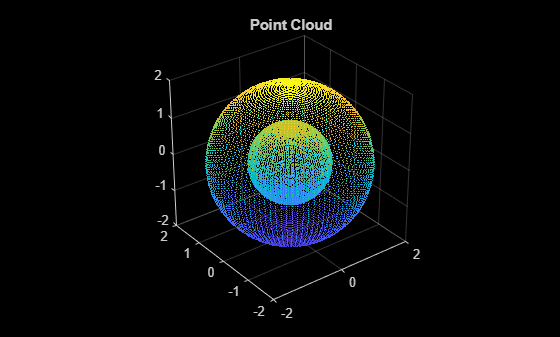# pcsegdist

Segment point cloud into clusters based on Euclidean distance

## Syntax

``labels = pcsegdist(ptCloud,minDistance)``
``[labels,numClusters] = pcsegdist(ptCloud,minDistance)``

## Description

````labels = pcsegdist(ptCloud,minDistance)` segments a point cloud into clusters, with a minimum Euclidean distance of `minDistance` between points from different clusters. `pcsegdist` assigns an integer cluster label to each point in the point cloud, and returns the `labels` of all points.```

example

````[labels,numClusters] = pcsegdist(ptCloud,minDistance)` also returns the number of clusters.```

## Examples

collapse all

Create two concentric spheres and combine them into a point cloud.

```[X,Y,Z] = sphere(100); loc1 = [X(:),Y(:),Z(:)]; loc2 = 2*loc1; ptCloud = pointCloud([loc1;loc2]); pcshow(ptCloud) title('Point Cloud')```Set the minimum Euclidean distance between clusters.

`minDistance = 0.5;`

Segment the point cloud.

`[labels,numClusters] = pcsegdist(ptCloud,minDistance);`

Plot the labeled results. The points are grouped into two clusters.

```pcshow(ptCloud.Location,labels) colormap(hsv(numClusters)) title('Point Cloud Clusters')```Load an organized lidar point cloud named `ptCloud`.

```load('drivingLidarPoints.mat') pcshow(ptCloud) title('Unclustered Point Cloud')```Detect the ground plane and store the points in `inliers`. Distance is measured in meters.

```maxDistance = 0.3; referenceVector = [0,0,1]; [~,inliers,outliers] = pcfitplane(ptCloud,maxDistance,referenceVector);```

Cluster the points, ignoring the ground plane points. Specify a minimum Euclidean distance of 0.5 meters between clusters.

```ptCloudWithoutGround = select(ptCloud,outliers,'OutputSize','full'); distThreshold = 0.5; [labels,numClusters] = pcsegdist(ptCloudWithoutGround,distThreshold);```

```numClusters = numClusters+1; labels(inliers) = numClusters;```

Plot the labeled results. Display the ground plane in black.

```labelColorIndex = labels+1; pcshow(ptCloud.Location,labelColorIndex) colormap([hsv(numClusters);[0 0 0]]) title('Point Cloud Clusters')```## Input Arguments

collapse all

Point cloud, specified as a `pointCloud` object.

Minimum Euclidean distance between points from two different clusters, specified as a positive scalar.

Data Types: `single` | `double`

## Output Arguments

collapse all

Cluster labels, returned as one of the following.

• If the point cloud, `ptCloud`, stores point locations as an unorganized M-by-3 matrix, then `labels` is an M-by-1 vector.

• If the point cloud, `ptCloud`, stores point locations as an organized M-by-N-by-3 array, then `labels` is an M-by-N matrix.

Each point in the point cloud has a cluster label, specified by the corresponding element in `labels`. The value of each label is an integer from `0` to the number of clusters of valid points, `numClusters`. The value `0` is reserved for invalid points, such as points with `Inf` or `NaN` coordinates.

Number of clusters, returned as a positive integer. The number of clusters does not include the cluster corresponding to invalid points.